# Reverse the Order of a List / Range – Excel & Google Sheets

This tutorial will teach you how to reverse the order of a list or range in Excel & Google Sheets.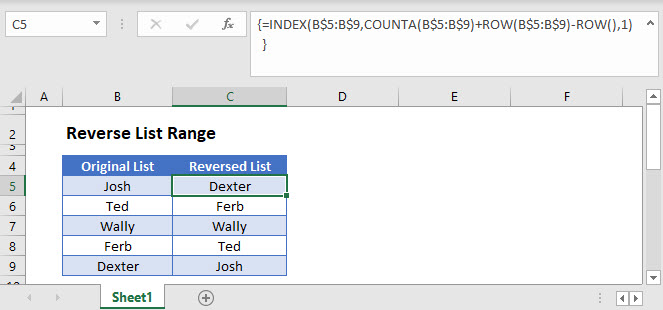## Reverse Order using INDEX Function

The INDEX function returns the value (can be any data type) positioned at the intersection of a specified row and column in a range or array. Its syntax is: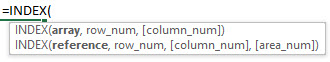We can use the INDEX function to reverse the order of a list of items like this:

``=INDEX(B\$3:B\$7,C3,1)``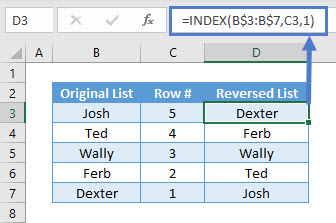In this example, column C provides the values for the ‘row_num’ argument of the INDEX function syntax. It has been calculated using the COUNTA and the ROW function, like this:

``=COUNTA(B\$3:B\$7)+ROW(B\$3:B\$7)-ROW()``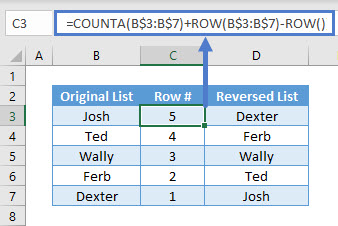## Reverse Order using INDEX, COUNTA & ROW Function

In the previous example, we split the formulas up to make it easier to follow. Instead, you can merge these formulas into one, like this:

``=INDEX(B\$3:B\$7,COUNTA(B\$3:B\$7)+ROW(B\$3:B\$7)-ROW(),1)``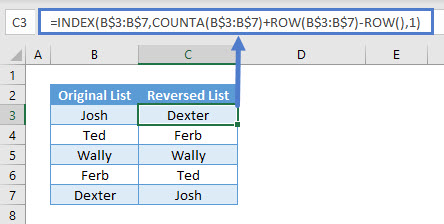Note: Mixed cell reference has been used for the range in each of the formulas to make it easier to drag down.

## Reverse the Order of a List / Range in Google Sheets

These formulas work exactly the same in Google Sheets as in Excel.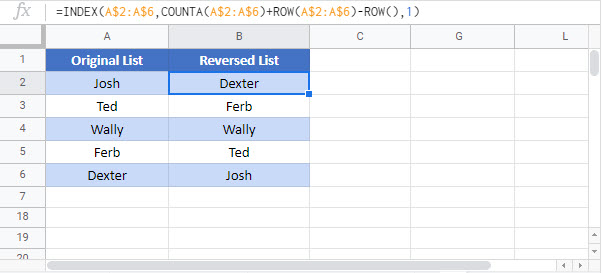### Excel Practice Worksheet

Practice Excel functions and formulas with our 100% free practice worksheets!